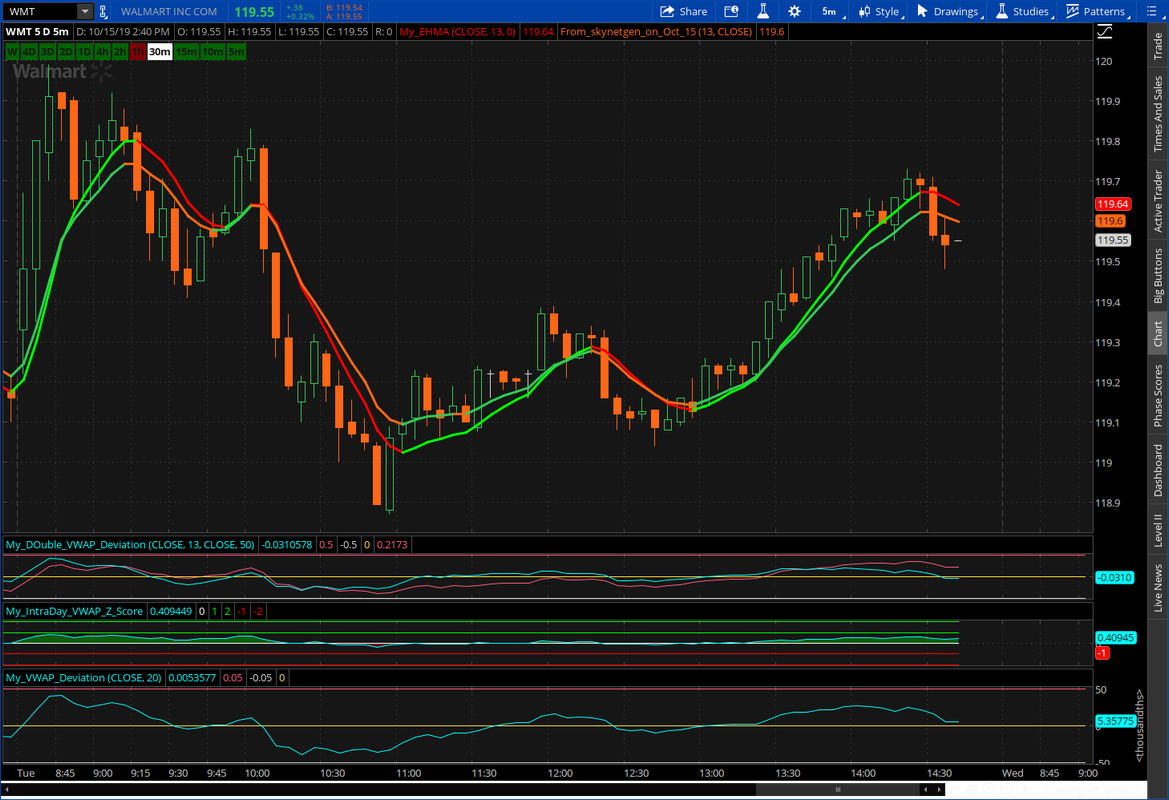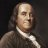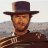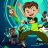# Exponential Hull Moving Average (EHMA) for ThinkorSwim

S

#### skynetgen

##### Well-known member
There was a study somewhere which said that exponential hull ma is best. Here is EHMA for TOS, converted from tradingview.

Code:
``````#skynetgen
declare upper;
input length =13;
input price = close;

script ehma{
input price=close; input length=13;
#HMA = WMA(2*WMA(n/2) − WMA(n)),sqrt(n))
#EHMA = EMA(2*EMA(n/2) − EMA(n)),sqrt(n))
plot ehma =
expaverage((2 * expaverage(price, length / 2))-expaverage(price, length / 2),
round(sqrt(length)));
};

plot MVA =ehma(price,length);

MVA.DefineColor( "up", Color.UPTICK );
MVA.DefineColor( "dn", Color.DOWNTICK );
MVA.DefineColor( "def", Color.PLUM );

MVA.AssignValueColor(
if MVA > MVA then MVA.Color( "up" )
else if MVA < MVA then MVA.Color( "dn" )
else MVA.Color( "def" ) );``````
https://tos.mx/Z7psKFb

p.s. Dont see anything special about it. yet Another MA.

Last edited by a moderator:
•Hguru#### horserider

##### Well-known member
VIP
Hmmm I tried to write an EHMA just from the formula. Seems it is different. Need to look at it closer, then will have more info.#### horserider

##### Well-known member
VIPS

#### skynetgen

##### Well-known memberInteresting. What is the formula you are using?#### horserider

##### Well-known member
VIP
Could be rounding up the values. Could be I did it incorrectely.Code:
``````# Exponential Hull Moving Avg by Horserider

input price = close;
input length = 100;
input displace = 0;

def halflength = Ceil(length / 2);
def sqrtlength = Ceil(Sqrt(length));
def val = 2 * ExpAverage(price, halflength) - ExpAverage(price, length);

plot HMA = ExpAverage(val, sqrtlength)[-displace];

HMA.SetLineWeight(2);
HMA.DefineColor("UP", Color.GREEN);
HMA.DefineColor("DOWN", Color.RED);

HMA.AssignValueColor(if HMA > HMA then HMA.Color("Up") else HMA.Color("Down"));``````

S

#### skynetgen

##### Well-known member
Could be rounding up the values. Could be I did it incorrectely.Well it is precisely because of rounding. all in all I dont really see anything special about either of these.

R

#### RascalDog

##### New member
These two versions both give later signals than the regular Hull MA.

S

#### skynetgen

##### Well-known member
These two versions both give later signals than the regular Hull MA.
Sometimes its not about late, but about less false signals. but overall I dont really see point to use it over regular HMA, considering its already built-in into TOS and supported by default

•markos
R

#### RascalDog

##### New member
Sometimes its not about late, but about less false signals. but overall I dont really see point to use it over regular HMA, considering its already built-in into TOS and supported by default
In the limited research I did, the late signals would have kept me out of the trade. I agree though that there is no point not to just use the TOS HMA.Hull moving average using data created with 9 period exponential MACD line ? Questions 11ATR and Exponential Moving Average Divergence Strategy (Ideal for Options) Strategies 33-in-1 Simple or Exponential Moving Average for ThinkorSwim Indicators 42